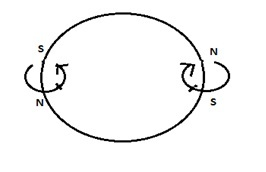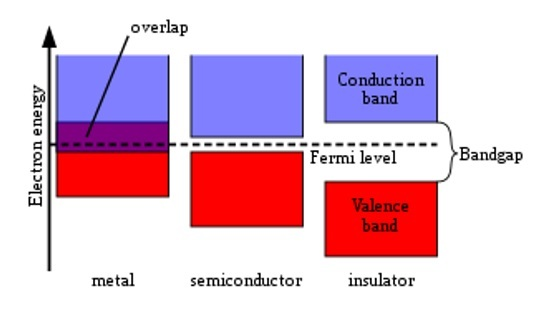# Band Theory of Solids

## Introduction

Band theory of Solids is essential to understand how energy is distributed in a solid. A huge number of atoms attached closely together is called Solids. Isolated atoms are initiated closer and closer simultaneously to construct solid. Valence electrons in a crystal are grouped into a single structure for the whole crystals. Because outermost electrons are interconnected with the orbits. Now electrons have to fill up the various energy levels. Atomic energy level is divided into a huge number of jointly spaced energy levels because of the electric force acted on each electron. Energy Band is a group of firmly spaced energy ranges.## What is Band Theory?

Energy levels of electrons, moments of electrons and state of electrons is derived theoretically in band theory. In a solid, every atom has a separate energy level. It is represented as 1s,2s,2p............... Every atom in the solid must be matching programmes to their energy level to become isolated. In an isolated atom, there are certain situations to be followed by electrons.

• Each atom consists of particular energy levels around them. Electrons cannot fill up in these energy levels.

• Lowest energy level is filled by electrons earlier. Quantum energy helps to push the electrons to the next range.

## What is the Band Theory of Solids?

In solids, atoms are closely packed. When two atoms are brought close together, their exterior energy level experiences a force from the nucleus of other atoms, and they get affected. If two-electron has the same energy level in the atom, then there is the formation of groups of energy level known as band. Hence, the study of this band in solids is known as the band theory of solids

## Why is the use of Band Theory?

Band theory is responsible for the optical property of the atoms in the solids. Conductors, Semiconductors, and insulators are based on electrical properties and the energy level of atoms. These three materials are more important for electrical conductivity.

### Conductors

Solids that have a huge amount of electrical conductivity are called Conductors. It happens in two methods. In the first method, a partially filled valence band. In the second method, a full valence band flaps the conduction band. So, the forbidden gap is zero. Hydrated Solutions of salts are good examples of conductors. For example, we take Sodium atoms (Na).

If an electric field is applied between solid sodium, high energy is obtained by the electrons. The energy acquired is in the form of kinetic energy, so the electrons move to form an electric current. So, the best example for conductors is sodium.Pieter Kuiper, Isolator-metal, marked as public domain, more details on Wikimedia Commons

### Semiconductors

If the electrical conduction of the material lies between the electrical conduction of the conductor and insulator, it is called Semiconductor materials. The energy gap of a semiconductor is 2 to 3eV or less than that. At room temperature, semiconductors conduct electricity more effectively than insulators. Examples of a semiconductor are Silicon and Germanium.

The conductivity of silicon is very low at low temperatures. But at a normal room temperature, a small number of electrons in the valence band holds enough kinetic energy to enter the conduction band after crossing the energy gap. When the electric field is applied, a limited amount of current passes through these electrons. The electrical resistivity of silicon lies between conductors and insulators. So. silicon metals are good semiconductors.

### Insulators

Materials that do not have electrical conduction are called insulators. The Energy Gap of insulators is more than 3 eV. At zero temperature in a diamond material, the conduction band will be empty and the valence band is filled up completely. It is too large. So, every electron is provided by 6 eV of energy. When an electric field is applied, there are no movements for electrons in the valence band. Because there is no unfilled level in this band. In solids, electrons do not accept these amounts of energy. So, in diamond crystals, electrons do not obtain the additional kinetic energy. So, diamond crystals will be an insulator.

## Solid Classification on the basis of Band Theory

Energy band structure determines the state of a solid whether it will be an insulator, conductor, or semiconductor, the arrangements of electrons with respect to these bands, and the width of forbidden bands.

Any material whose electrical conduction is enormous is called a conductor. If the electrical conduction of the material lies between the electrical conduction of the conductor and insulator, it is called Semiconductor materials. Materials that do not have electrical conduction are called insulators.

### Valence Band

A band that is filled with electrons fully or partially in the outermost band is called the valence band. High energy is kept in these electrons. Valence bands are formed by valence electrons. Valence bands consist of a group of states containing the outermost or the valence electron of an atom. Electrical, thermal, and optical properties of solids are depending on valence bands.

### Conduction Band

The next higher allowed energy band which is above the valence band is known as the Conduction band. The valence band is separated from a conduction band by a small gap.

The gap between valence and conduction band replaces the energies that their electron cannot have. The conduction band is parallel to the first high states and is normally the smallest unfilled energy band. Conduction electrons are free electrons which are moving freely on conduction bands. At room temperature, it has conduction electrons or free electrons which conduct heat and electricity.

### Forbidden Band

The electrons can gain only the energy values which are in the band. The gap between the two allowed bands is called the Forbidden band. Energy bands of atoms are divided by ranges, which have no allowed energy levels. This range is known as forbidden bands.

## Conclusion

Band theory is the theoretical explanation of energy levels or the energy range of electrons in the solids. Band theory is also responsible for the optical and electrical properties of Solids. A huge number of atoms attached closely together is called Solids. Isolated atoms are initiated closer and closer simultaneously to construct a solid. There are three types of bands in the solids. Valence band, conduction band, and forbidden band. Also based on the energy level of electrons in atoms of the solids, it is divided into semiconductors, conductors, and insulators.

## FAQs

Q1. What are Superconductors?

Ans. Materials that conduct electricity without resistance are called superconductors. In this state, the material has zero resistivity. This property is called as superconductivity.

Q2. What is the bandgap?

Ans. Bandgap is the net distance at which electrons can take up energy values in that range regardless of the direction of their motion.

Q3. Define conductor and Semiconductor on the basis of their electrical conductivity.

Ans. In conductors the electrical conductivity is decreased with respect to addition of impurities and increase of temperature due to decrease in mean free path. In Semiconductors the electrical conductivity is increased with respect to addition of impurities and increase of temperature due to increase of charge density.

Q4. What are the optical properties of Solids?

Ans.

• Not all metals are translucent to light of all wavelengths.

• Insulators are crystal clear to visible lights.

• Semiconductors are transparent to optical light but non transparent to visible light.

Q5. What are the properties of solids which are related to dielectric constant?

Ans. Some solids are transparent and some opaque to the light. Some solid surfaces are highly reflecting. But solid surfaces bend the electromagnetic waves incident on them. These properties have a close relationship with the dielectric constant ϵ(ω).

Updated on: 18-Apr-2023

2K+ Views